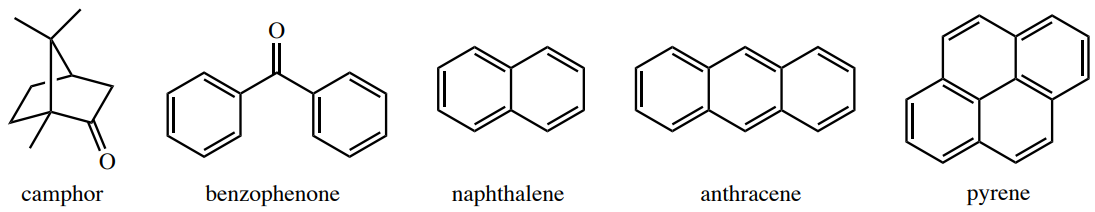# 6.3A: Overview of Sublimation

•• Lisa Nichols
• Butte College
$$\newcommand{\vecs}{\overset { \rightharpoonup} {\mathbf{#1}} }$$ $$\newcommand{\vecd}{\overset{-\!-\!\rightharpoonup}{\vphantom{a}\smash {#1}}}$$$$\newcommand{\id}{\mathrm{id}}$$ $$\newcommand{\Span}{\mathrm{span}}$$ $$\newcommand{\kernel}{\mathrm{null}\,}$$ $$\newcommand{\range}{\mathrm{range}\,}$$ $$\newcommand{\RealPart}{\mathrm{Re}}$$ $$\newcommand{\ImaginaryPart}{\mathrm{Im}}$$ $$\newcommand{\Argument}{\mathrm{Arg}}$$ $$\newcommand{\norm}{\| #1 \|}$$ $$\newcommand{\inner}{\langle #1, #2 \rangle}$$ $$\newcommand{\Span}{\mathrm{span}}$$ $$\newcommand{\id}{\mathrm{id}}$$ $$\newcommand{\Span}{\mathrm{span}}$$ $$\newcommand{\kernel}{\mathrm{null}\,}$$ $$\newcommand{\range}{\mathrm{range}\,}$$ $$\newcommand{\RealPart}{\mathrm{Re}}$$ $$\newcommand{\ImaginaryPart}{\mathrm{Im}}$$ $$\newcommand{\Argument}{\mathrm{Arg}}$$ $$\newcommand{\norm}{\| #1 \|}$$ $$\newcommand{\inner}{\langle #1, #2 \rangle}$$ $$\newcommand{\Span}{\mathrm{span}}$$$$\newcommand{\AA}{\unicode[.8,0]{x212B}}$$

Some compounds are capable of sublimation, which is the direct phase change from solid to gas. Solid carbon dioxide is an example of a substance that sublimes readily at atmospheric pressure, as a chunk of dry ice will not melt, but will seem to "disappear" as it turns directly into carbon dioxide gas. Sublimation is an analogous process to boiling, as it occurs when a compound's vapor pressure equals its applied pressure (often the atmospheric pressure). The difference is that sublimation involves a solid's vapor pressure instead of a liquid's. Most solids do not have an appreciable vapor pressure at easily accessible temperatures, and for this reason the ability to sublime is uncommon. Compounds that are capable of sublimation tend to be those with weak intermolecular forces in the solid state. These include compounds with symmetrical or spherical structures. Examples of compounds that can be sublimed are in Figure 6.28.Figure 6.28: Compounds that can be vacuum sublimed.$$^8$$

As relatively few solids are capable of sublimation, the process can be an excellent purification method when a volatile solid is contaminated with non-volatile impurities. The impure solid is heated in the bottom of a vessel in close proximity to a cold surface, called a "cold finger" (Figure 6.29). As the volatile solid sublimes, it is deposited on the surface of the cold finger (where it can later be recovered), and is thus separated from the non-volatile substance left in the vessel. Sublimation is an example of a "green chemistry" technique, as no solvents are used and no waste is generated. The process, however, is not particularly efficient at separating volatile solids from one another.

Of the solids with appreciable vapor pressures at room temperature, many still require rather high temperatures to actively sublime (when their vapor pressure equals the atmospheric pressure of nearly $$760 \: \text{mm} \: \ce{Hg}$$). If these solids are heated to their sublimation points under atmospheric pressure, some will char and decompose during the process. For this reason, it is very common to perform sublimation under a reduced pressure (vacuum sublimation). Analogous to vacuum distillation in which liquid boils when its vapor pressure equals the reduced pressure in the apparatus, in vacuum sublimation solid sublimes when its vapor pressure equals the reduced pressure in the apparatus. In vacuum distillation, reducing the pressure allows for liquids to boil at a lower temperature. Similarly, reducing the pressure in vacuum sublimation allows for solids to sublime at a lower temperature, one which avoids decomposition.
$$^8$$As reported in D. D. Perrin, W. L. F. Armarego, Purification of Organic Chemicals, Pergamon Press, 3$$^\text{rd}$$ edition, 1988.